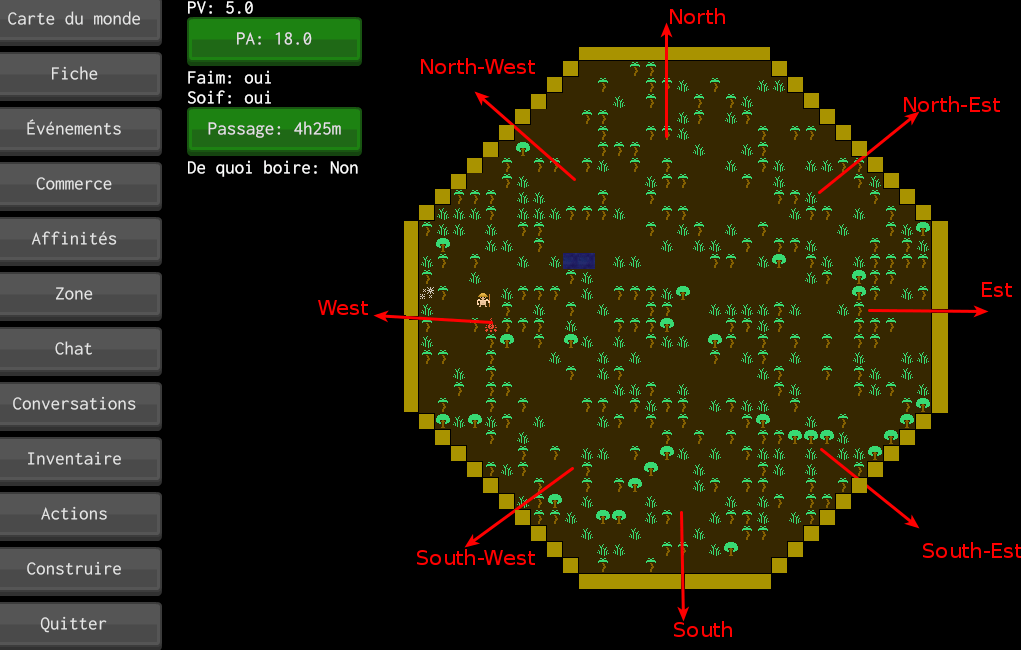## #StackBounty: #mathematics #tiles Octagon border algorithm

### Bounty: 50

I work on an open source game since 2 years and I’m very bad at math (it is not every time easy haha). My game permit to move a character on octagon. When character reach border coordinate (colored in yellow), I permit him to travel on a “new octagon”:So, the algorithm goal is to know if an x, y tile coordinate is on a “yellow” tile, which direction is (North or North-Est or Est …) depending on map width and height.

I wrote this algorithm many times and in two different language, example with Rust:

``````pub fn get_corner(width: i16, height: i16, new_row_i: i16, new_col_i: i16) -> Option<CornerEnum> {
let left_col_i_end = width / 3;
let right_col_i_start = (width / 3) * 2;
let top_row_i_end = height / 3;
let bottom_row_i_start = (height / 3) * 2;
let mut more = if new_row_i >= 0 { new_row_i } else { 0 };
#[allow(unused_assignments)]
let mut right_col_i = 0;
#[allow(unused_assignments)]
let mut left_col_i = 0;

if new_row_i < top_row_i_end {
right_col_i = right_col_i_start + more;
left_col_i = left_col_i_end - more;
} else {
if new_row_i >= bottom_row_i_start {
more = (height / 3) - (new_row_i - bottom_row_i_start + 1);
more = if more >= 0 { more } else { 0 };
right_col_i = right_col_i_start + more;
left_col_i = left_col_i_end - more;
} else {
left_col_i = left_col_i_end;
right_col_i = right_col_i_start;
}
}

if new_col_i < left_col_i && new_row_i < top_row_i_end {
return Some(CornerEnum::TopLeft);
}
if new_row_i < 0 && left_col_i <= new_col_i {
return Some(CornerEnum::Top);
}
if new_col_i >= right_col_i && new_row_i < top_row_i_end {
return Some(CornerEnum::TopRight);
}
if new_col_i > width - 1 && top_row_i_end <= new_row_i {
return Some(CornerEnum::Right);
}
if new_col_i >= right_col_i && new_row_i >= bottom_row_i_start {
return Some(CornerEnum::BottomRight);
}
if new_row_i > height - 1 && left_col_i_end <= new_col_i {
return Some(CornerEnum::Bottom);
}
if new_col_i < left_col_i && new_row_i >= bottom_row_i_start {
return Some(CornerEnum::BottomLeft);
}
if new_col_i < 0 && top_row_i_end <= new_row_i {
return Some(CornerEnum::Left);
}

None
}
``````

But it is not working well … I curse my math. I’m sure it’s not that complicated but i fail at each time in two years. So, i’m here to ask help, or for solution. That would be greatly appreciated!

Get this bounty!!!